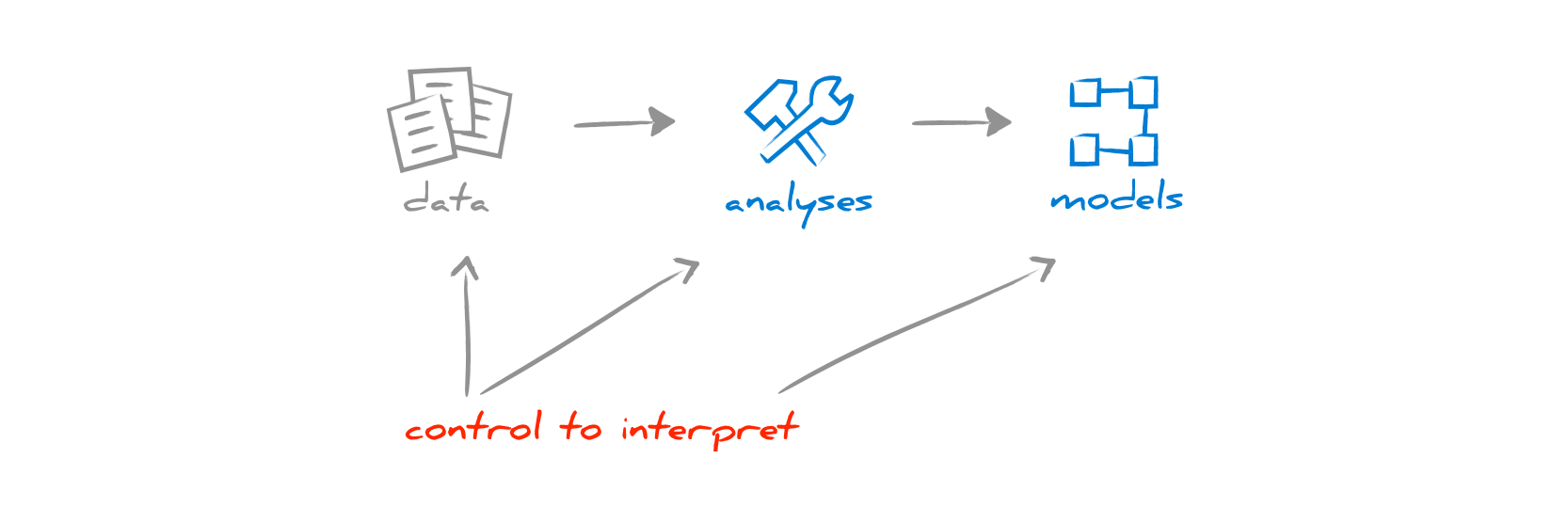# Analysis anatomy

To take control of analyses, we first need to understand their inner nature.

## Analysis in a nutshell

Analyses can be complex at times, but their essence is actually not complicated. Conceptually, all analyses share a simple organization. They all require an input set of data, they have an algorithm of some sort, and produce a model that represents a certain subset of the characteristics of the input data.

This applies to any kind of analysis. For example:

• a metric transforms the input data into a number, or
• a visualization transforms the input data into a picture.

Of course, in a larger analysis, there can be a multitude of such transformations. For example, consider a visualization displaying entities enriched with metrics: first the entities need to be extracted, then the metrics are computed, and finally the picture is put together.

## Control to interpret

The goal of analysis is to provide a summary to ease the understanding of the original data. But, to be able to interpret the result of an analysis you need to control both the input data and the decisions taken by the analysis algorithm.Let us consider a simple example of measuring the size in terms of number of methods of the following class:

```public class Library {
List books;
public Library() {…}
public void removeBook(Book b) {…}
private boolean hasBook(Book b) {…}
protected List getBooks() {…}
protected void setBooks(List books) {…}
public boolean equals(…) {…}
}```

How many methods are there? 7. But, is a constructor a method? If the metric computation does not consider it as a method, we get 6 instead of 7. What about setters and getters? Are they to be considered as methods? If no, we have only 4. Do we count the private methods as well? Perhaps the metrics is just about the public ones. In this case, the result is only 3. Finally, `equals()` is a method expected by Java, so we might as well not consider it a real method. So, perhaps the result is 2.

How many methods are there? All these are valid answers depending on what we understand by the question.

Now, let's turn the situation around, and consider a report says a class has 70 methods. What does it mean? You have to know what the actual computation does.

But, wait. This is still not enough.

Let us consider another example of computing the size of an entire system in terms of the total number of methods from all system classes. Suppose that we know that the number of methods metric answers 7 for the above example, and that the result is 20'317 for the entire system. This number does not yet have an interpretation unless we know what "all system classes" entails. Were generated classes included in this set? How about the classes from the third party frameworks?

If you want to be able to interpret the result of applying an analysis, you need to know both what the input set of data was, and what the algorithm does.